Liu, H., Dong, J., Jing, H., Guo, X., Duan, C., Qi, K., Yang, R., Guo, H., Wang, B., and Qiao, L. (2020). "Refining characteristics of isometric straight bar plates with different bar angles," BioRes. 15(4), 7844-7860.

#### Abstract

Bar angle is one of the most important parameters of an isometric straight bar refiner plate and its effects on the change in pulp and paper properties during refining is still unclear. This study investigated the effect of plate bar angle on refining quality and refining efficiency by clarifying the definition of bar angle. Five isometric straight bar plates with different bar angles were used in low consistency refining of bleached sulphate eucalyptus pulp (BSEP). Samples at different refining time intervals were collected, and the fibers, pulp, and paper properties were detected and analyzed. There was a great influence of bar angle on the fibers, pulp, and paper properties. Additionally, a critical static attack angle, or critical bar angle for plates with specific field angle, existed. The modification of fibers and pulp properties was strong when the plate reached a critical bar angle. Meanwhile, there was an optimal static attack angle for a plate with specific field angle, when considering paper properties such as tensile index and tear index. The refining time was shortest when the pulp was refined by the plate with critical bar angle, allowing the paper properties to reach a maximum value. It was shown that the static attack angle of refining should be selected in the range of 45º to 70º, which includes the optimal value and the bar angle was proposed in the range of 11.25º to 23.75º for the plate with common field angle of 22.5º.

Refining Characteristics of Isometric Straight Bar Plates with Different Bar Angles

Huan Liu,a,b,* Jixian Dong,a,b,* Hui Jing,a,b Xiya Guo,a,b,c Chuanwu Duan,a,b Kai Qi,a,b Ruifan Yang,a Haozeng Guo,a,b Bo Wang,a,b Lijie Qiao a,b

Bar angle is one of the most important parameters of an isometric straight bar refiner plate and its effects on the change in pulp and paper properties during refining is still unclear. This study investigated the effect of plate bar angle on refining quality and refining efficiency by clarifying the definition of bar angle. Five isometric straight bar plates with different bar angles were used in low consistency refining of bleached sulphate eucalyptus pulp (BSEP). Samples at different refining time intervals were collected, and the fibers, pulp, and paper properties were detected and analyzed. There was a great influence of bar angle on the fibers, pulp, and paper properties. Additionally, a critical static attack angle, or critical bar angle for plates with specific field angle, existed. The modification of fibers and pulp properties was strong when the plate reached a critical bar angle. Meanwhile, there was an optimal static attack angle for a plate with specific field angle, when considering paper properties such as tensile index and tear index. The refining time was shortest when the pulp was refined by the plate with critical bar angle, allowing the paper properties to reach a maximum value. It was shown that the static attack angle of refining should be selected in the range of 45º to 70º, which includes the optimal value and the bar angle was proposed in the range of 11.25º to 23.75º for the plate with common field angle of 22.5º.

Keywords: Pulp refining; Disc refiner; Isometric straight bar plate; Bar angle; Refining characteristics

Contact information: a: College of Mechanical & Electrical Engineering, Shaanxi University of Science & Technology, Xi’an, Shaanxi Province, 710021 People’s Republic of China; b: China Key Laboratory of Light Industry Equipment Manufacturing Intelligence, Xi’an, Shaanxi Province, 710021 People’s Republic of China; c: College of Art & Design, Shaanxi University of Science &Technology, Xi’an, Shaanxi Province, 710021 People’s Republic of China;

* Corresponding authors: liuhsust@126.com; djx@sust.edu.cn

INTRODUCTION

Refining is an energy-intensive operation in the pulp and papermaking industry. Pulp flows in the refining zone between the rotor and stator surface of a disc refiner and is subjected to complex forces induced by the refining plates and fluid mechanics. As a consequence, the pulp or fiber properties, such as internal fibrillation, external fibrillation, fiber cutting, fines, fiber curl, as well as fiber morphology, are changed, which strongly effect the properties of formed paper (Giertz 1980; Ebeling 1980; Page 1989).

Many factors are able to affect the refining quality, such as the plate gap, rotation speed, and plate bar profile. The refining plate is one of the most important components in refining as it directly applies mechanical forces on pulp or fibers and its bar profile has a strong influence on the pulp quality when the gap and rotation speed are held constant. In previous studies, the effect of bar height and width on the refining process have been explored. The pulp quality was better and energy consumption was lower when refining with higher bar height plates than that with lower bar height (Li and Ma 1995). Meanwhile, there was a slight influence of bar width on hardwood pulp during medium consistency refining, and the freeness drop was slow when refining using a wider bar width plate (Liu and Chen 2006, 2007). Different configurations of bar width and groove width can affect the refining intensity and characteristics during an otherwise similar refining process; this was explored by Lundin et al. (1999) and Elahimehr et al. (2015). In addition to the individual bar parameters, changes in the bar structure can directly affect the refining intensity and thus the refining quality and efficiency (Yang and Jiang 2010; Elahimehr et al. 2015; Dino et al. 2018).

Straight bar plates are widely used in pulp refining due to their convenient design and various bar structures, compared to the curved bar plates. The isometric straight bar plate is a type of straight bar plate that is commonly used in low consistency refining. There are two main groups of isometric straight bar plate, one stage and multi-stage isometric straight bar plates (Liu et al. 2020), as shown in Fig. 1. The bar width and groove width of (a) keep constant in the refining zone, however, that of (b) in the same stage keep constant and the bar and groove width among different stages may be different.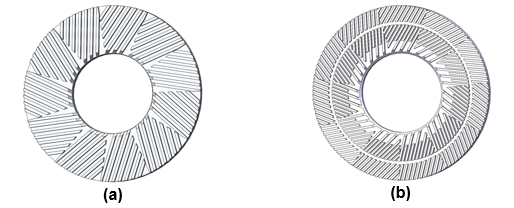Fig. 1. Two types of isometric straight bar plate, one stage (a) and multi-stage (b) isometric straight bar plates

Bar angle is an important parameter of isometric straight bar plates measuring for the inclination degree of the bar, and its direction and size have great influence on pulp flow, no-load power, refining quality, and efficiency (Xie 2015). At present, no uniform standard of bar angle definition has been proposed; usually the angle between bar and radius direction is in the range 15º to 20º (He 2010). When the bar angle is zero, all bars are distributed radially, and the direct interleaving of rotor and stator bars has a strong cutting action on fibers and a noisy refining process, which means that it is necessary to set a reasonable bar angle (Chen and Chen 2010 ). When the specific energy consumption of refining is held constant, the pulp freeness will be affected by the plate bar angle (Siewert and Selder 1980). The refining time to obtain a given pulp freeness and energy consumption can be reduced when refining with a smaller bar angle plate (Brecht et al. 1965). As plate bar angle increases, the fiber cutting effect is smaller and aspect ratio of fiber increases (Liu 2014). In addition, the bar angle direction will affect the characteristics of pulp flow during refining. There is a pumping effect on pulp when bars tend to be opposite to the rotation direction of rotor, and pump capacity is stronger when the bar angle is larger. A larger bar angle induces a smaller fiber residence time and a higher yield; however, there is a sharp drop in overall pulp quality (Vomhoff 1990; Xie 2015).

Bar interaction is the basic premise of the impact of refining plates on fibers. The primary parameters of bar interaction are the number of bar interaction points, the bar interaction area, and the bar interaction length (Elahimehr et al. 2015; Liu et al. 2019). The bar angle will affect bar interaction parameters, which directly affect the pulp quality and energy consumption. Kenichi Ito et al. (2006) found that bar attack angle generally ranges between 15º and 40º, with an average attack angle of 30º, when refining by a straight bar plate with bar angle of 10º. However, Grzegorz and Dariusz (2013) considered that the bar angle is half of the attack angle, but they did not introduce a definition for bar angle. The determination of bar attack angle is important for the improvement of refining quality and efficiency. Chen et al. (2010) considered the best bar attack angle is approximately 20º through 37º, while Sigl (1999) considers the best attack angle to be 40º by comparing the refining characteristics of two plates with bar angles of 60º and 40º. Up to now there have been few experimental trials on bar attack angle, and further research is needed to fully document this phenomenon. Few previous studies that systematically researched bar angle and attack angle of isometric straight bar plate and its effect on refining quality were found, and no clear definition of bar angle as well as the in-depth study of them have been clarified. To explore the influence of bar angle on pulp quality and refining efficiency, five series of low consistency refining trails were conducted using five one stage isometric straight bar plates (hereinafter collectively referred to as isometric straight bar plates) with different bar angles by clearly defining the bar angle and static attack angle. The effects of bar angle on fiber, pulp, and formed paper properties were analyzed. The mechanism of how bar angle applies impact on fibers and their optimal range were initially determined, which is beneficial to the design and selection of refining plates.

EXPERIMENTAL

Methods

Bar angle and static attack angle of straight bar plate

The angle between the bar and the radial direction is usually called the bar angle, as shown in Fig. 2 (a). The bar angle of the different bars in a bar field, composed of a single cluster of bars, varies with the position of the bars, such as α1-1 and α1-i, which is not conducive to characterizing the bar angle of the segment of the refining plate.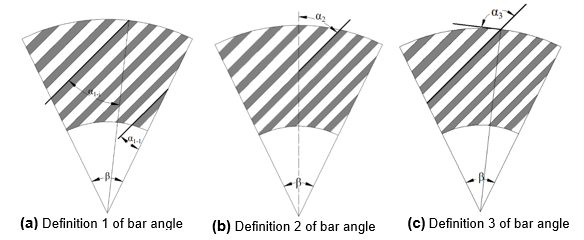Fig. 2. Definition of isometric straight bar plate (or segment) bar angle

Currently, there are three main definitions of the bar angle of the isometric straight bar plates or segments. Firstly, the bar angle is defined as the angle α1-1 between the first bar and the right edge of the single cluster bars field, as shown in Fig. 2(a) (Roux et al. 2011). Secondly, the degree of bar inclination can be measured by the angle α2 between the center axis of the field and bar, as shown in Fig. 2(b) and the last one αas shown in Fig. 2(c) (Chen et al. 2010).

The bar angle α1-1 and the field angle β of the segment directly affect the bar angle α2, which means that the nature of α1-1 and α2 is the same for measuring the angle of the segment for a specific field angle β (Liu et al. 2019). However, the bar angle α1-1 would be easily used due to convenient measurement, and it was used in this paper to measure the bar inclination.

According to the refining plate (or segment) used in actual refining, it can be roughly classified into left-handed and right-handed plates by considering the direction of bar angle related to horizontal line. As shown in Fig. 3, the refining segment with the field angle of β was cut by the horizontal line l, and the angle between line l and edge of field is the critical bar angle αc, which can be expressed as Eq. 1,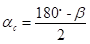(1)

where αc is the critical bar angle (°) of the left-handed and right-handed plate, when α is less than αc, the refining plate is a right-handed plate; when α is greater than αc, it is a left-handed plate.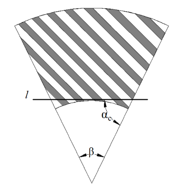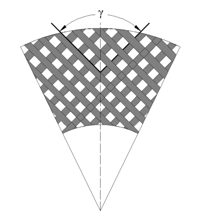Fig. 3. Critical bar angle for left-handed and right-handed plates Fig. 4. Static attack angle of isometric straight bar plate

The static attack angle γ reflects the angle between the rotor bars and stator bars during the actual refining process, which can be defined as the angle between the bars of rotor and stator when two plates are relatively stationary, as shown in Fig. 4. For straight bar plates, the actual attack bar angle is in the process of dynamic change during refining; however, the static attack angle can vary relative to the value of the bar angle when different bar angle plates are considered. It can be expressed by Eq. 2: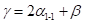(2)

It can be obtained from Eq. 2 that the size of the attack angle is affected by both the field angle and bar angle.

When the specific application of the refining plate is not considered, the static attack angle can be varied from 0º to 180º. Therefore, the design value range of the bar angle for the right-hand refining plate is given as Eq. 3: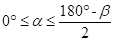(3)

However, the bar angle of an isometric straight bar plate should be reasonably selected in actual design according to the actual application requirements.

Characterization of refining process

The energy-based and force-based refining intensities, such as specific energy consumption, specific edge load (SEL), and specific surface load (SSL), are commonly used parameters to measure the refining process (Liu et al. 2018). As simple and easily calculated refining intensity, SEL, which combines the bar profile and process parameters of refining together, was used in this research to characterize the refining process. It can be expressed by Eq. 4,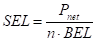(4)

where Pnet is the net power (kW), n is the rotation speed of rotor (rpm), and BEL is the bar edge length (km/r).

BEL of the straight bar plates with the same rotor and stator bar parameters can be calculated with Eq. 5 (Roux et al. 2009),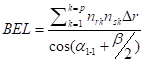(5)

where nrk and nsk are the bar number of rotor and stator in the ring zone k, △r is the width of ring zone k (mm).

In addition, the key variable for fiber shorting is force—not energy—as proposed by Kerekes and Meltzer (2018), so it would be an effective method to characterize refining by the bar force applied by the plates. Specific edge load can be obtained by Eq. 6,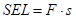(6)

where F is the bar force (N/m) and s is the distance of movement during which force is exerted.

Thus, the bar force F can be expressed by Eq. 7,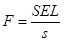(7)

when the conditions of bar width, W (mm), is larger than fiber average length, (mm), + 5 C l, and in the opposite case, + 5 C l, in which C is the pulp consistency.

Materials

Refining

In the refining trials, bleached sulphate eucalyptus pulp (BSEP) board was used as the raw material, and it was soaked in distilled water for 4 h and then dissipated by a pulp disintegrator PD10 (Techlab Systems, San Sebastian, Spain), and its consistency was adjusted to 3%. The MD-3000 single-disc experimental refiner (Regmed, Osasco, Brazil) was used, as shown in Fig. 5, and the refining trials were conducted using different plates at a constant speed of 1460 rpm. Five straight bar plates with different bar angle and related bar parameters were directly processed by Nantong Huayan Casting Co., Ltd. (Nantong, China) through the computer numerical control (CNC) machine tools (X6132; Shenzhen Dima Co., Ltd., Shenzhen, China). Table 1 shows the detailed bar parameters of them. According to the Eq. 3, the calculated bar angle for plates used in the trials was in the range of 0º to 70º; however, the actual bar angles were selected as 0º, 5º, 22º, 39º, and 50º, considering the flow rate and refining efficiency.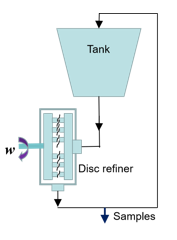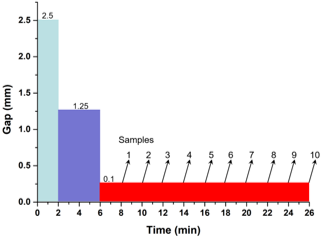Fig. 5. MD3000 disc refiner Fig. 6. The process conditions of refining trials

The process conditions of the refining trial are shown in Fig. 6, and 10 pulp samples were collected at a constant gap of 0.1 mm during different refining times. Considering the effect of plate gap on the pulp or fiber properties, the pulp with a gap clearance of 0.1 mm was sampled at intervals of 2 min. Then they were centrifuged and dried by an electric blast drying oven (101; Beijing Zhongxing Weiye Instrument Co., Ltd., Beijing, China) to calculate the moisture content of the pulp for subsequent experiments.

Table 1. Bar Parameters of Five Isometric Straight Bar Plates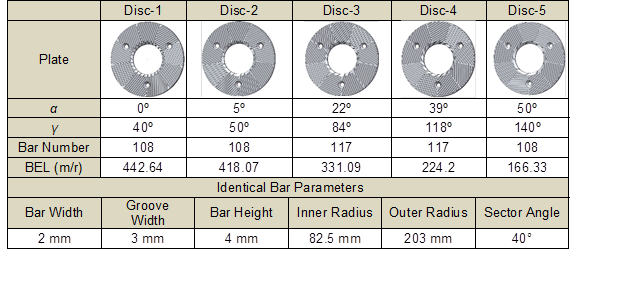Measurement of pulp, fiber, and paper properties

The average fiber length, fines content and fiber fibrillation of the pulp samples were analyzed by a FS5 (Valmet, Espoo, Finland) fiber quality analyzer. The scanning electron microscopy (SEM) analysis (S-4800; HITACHI, Tokyo, Japan) was conducted to observe the fiber morphology of the different samples. The pulp freeness was determined using a DFR-05 drainage freeness retention (BTG Group, Herrsching, Germany). Handsheets for measuring the physical properties of paper were made and their physical properties, including tensile and tear strength, were measured based on TAPPI T220sp-06 (2001) using a tensile strength meter 062 (L&W, Kista, Sweden) and a paper tear strength meter SLY-S1 (Labthink, Jinan, China).

RESULTS AND DISCUSSION

SEL and Bar Forces

The SEL and bar forces are two methods to measure the refining characteristics of pulp refining process. Bar force is only used in research while SEL is widely used not only in research but also in the industry. Both of them were used in this study to better characterize the refining process conducted with isometric straight bar plates with different bar angles.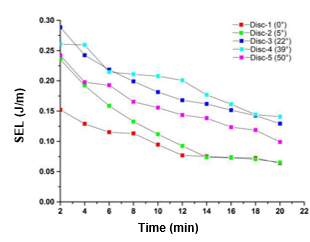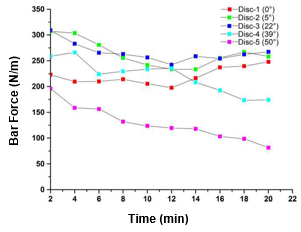Fig. 7. SEL of different refining processes using different bar angle plates over time Fig. 8. Bar force of different refining processes using different bar angle plates over time

The specific edge load, a typical measurement of the refining intensity, was affected by the net power, rotation speed, and characterization parameters of the refining plate (according to Eq. 4) during the refining process. It can be concluded that the BEL of a straight bar plate is larger when the bar angle is smaller, as shown in Table. 1. However, SEL gradually decreased as refining time increased, regardless of the bar angle of the straight bar plates, as shown in Fig. 7. Meanwhile, the value of SEL for isometric straight bar plates increased as the bar angle increased when the refining time was the same, except for a plate with a bar angle of 50º. In general, a refining process with low intensity produces more fiber fibrillation, less cutting, and higher sheet strength, while a higher refining intensity produces pulps with lower strength and increased fiber cutting (Stationwala et al. 1991; Muhić et al. 2011). Therefore, a greater degree of fibrillation and less cutting can be achieved during refining with the smaller bar angle isometric bar plate through the theoretical analysis of SEL.

Bar force is another key factor that influences the refining quality, especially when considering fiber shorting (Kerekes and Meltzer 2018), and it was adopted to measure the hardwood pulp refining, as shown in Fig. 8. In the authors’ trials, the bar force decreased with the change of power and fiber average length over refining time except for Disc-1. Meanwhile, the bar force of an isometric straight bar plate with a smaller bar angle was larger than that of the lager one, except Disc-1, and its difference between two plates with larger bar angle were more obvious especially for Disc-5. This means the cutting effect of the straight bar plate with a smaller bar angle was higher, while the fiber shorting of the straight bar plate with bar angle of 0º, was less than that of Disc-2. The conclusion obtained from theoretical analysis of bar force was consistent with Liu (2014) and the results of this research. However, the special result of Disc-1 should be further theoretically studied by combining the bar pattern and the dynamics of bar interaction.

Pulp Freeness

Drainage performance of pulp samples collected at intervals of 2 min, refer to a “zero point” when the gap was adjusted to 0.1 mm, was characterized by Canadian Standard Freeness (CSF) as per TAPPI T227 om-99 (1999), which comprehensively characterizes fiber cutting, fibrillation, and swelling.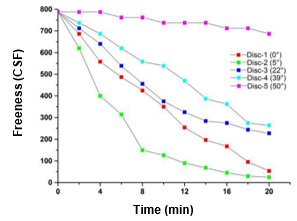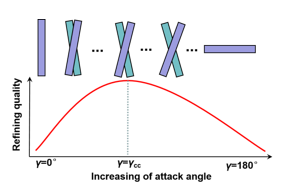Fig. 9. The pulp freeness obtained from different bar angle plates as a function of time Fig. 10. Critical attack angle and its effect on refining quality and efficiency

Figure 9 shows the freeness changes of the pulp obtained from the five different plates as a function of refining time. The pulp freeness gradually decreased with time, but its rate of change also decreased with increasing refining time. Meanwhile, the freeness of different pulp samples obtained at the same time but refined by different plates was different, which seems to show that it decreased with the increasing of the bar angle except for samples obtained from Disc-1. The reason for this may be because there is a critical bar angle αcc, or static attack angle γcc, that leads to the highest refining efficiency, as shown in Fig. 10. The ability of a refining plate to reduce freeness was lowered when the static attack angle was beyond γcc and increased when γ was less than γcc. The rate of change in freeness was calculated by considering the freeness of pulp samples that were refined for a total of 0 and 20 min. The rate of change in freeness for Disc-5 was 12.7%, which was smaller than that of Disc-2, 96.8%. Therefore, it can be concluded that there was less effect on pulp or fiber properties when refining using a plate with a bar angle of 50º and the refining process would be ineffective and enormously energy intensive. Through the analysis of freeness, the critical bar angle αc was in the range of 5º to 22º for the straight bar plates with field angle of 40º —which tended to be approximately 10º for the plate in this trial—and would allow the greatest degree of freeness reduction to be reached.

Fiber Properties

Fiber properties, such as fiber length, fines content, and fibrillation, are greatly affected by the bar profile of plates, which would further affect the properties of the formed paper through changing the bonding ability of fibers.

Fiber length and fines content

The length-weighted average fiber length, Ll, is one of the commonly used methods for measuring fiber length, and it was used in this work to analyze the influence of bar angle on fiber length during refining. As shown in Fig. 11, the average fiber length Ll was gradually reduced with increasing time due to the complex mechanical effects of the refining plates. However, there were obvious differences between the cutting ability of the refiner plates with different bar angles. A new parameter, fiber cutting rate, is described in this article, which references the change in fiber length relative to the original fiber length after refining for 20 min. The cutting rate of the plate with a bar angle of 5º on fibers was 49.7%, which was the largest among plates, while the smallest value was the cutting rate of Disc-5, 18.9%. It also can be concluded that a critical bar angle, or static attack angle, exists, and the fiber cutting effect of an isometric straight bar plate that with bar angle or static attack angle is stronger compared to all others, which is consistent with the conclusion of Fig. 10 and the prediction of bar force proposed by Kerekes and Meltzer (2018). It was found that the αcc would be in the range of 5º to 15º through the analysis of the variation of average length of fibers refined by straight bar plates with different bar angles, as shown in Fig. 12. However, the critical static attack angle, in the range of 50º to 70º, is more useful compared to critical bar angle αcc in the actual design of straight bar plates, because γcc is the production of αcc and β. Further, the optimal bar angle can be determined by considering the γcc and a reasonably designed β, aiming to improve the refining efficiency and reduce the energy consumption.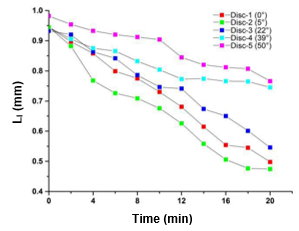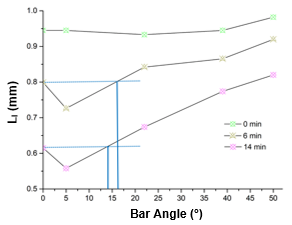Fig. 11. The length-weighted average fiber length Ll of the different pulp samples obtained by different plates over time Fig. 12. The relationship between the bar angle and the average fiber length at the same refining time

Fines content is a main factor that affects pulp drainage and paper properties and is greatly affected by operation parameters and the bar profile of plate. Flake-like fines, which are particles with a length of less than 0.2 mm, of pulp samples refined by different plates was quantitatively analyzed by a fiber quality analyzer, as shown in Fig. 13. The fines content increased when it was continuously refined due to fiber cutting and excessive external fibrillation. The value of fines content in pulp samples obtained by Disc-1, 2, and 3 was closer, but the fines content of Disc-2 was slightly higher than that of Disc-3. Meanwhile, the increasing rate of fines content of different bar angle plates was different, and it was 14.68% and 3.96% for Disc-4 and 5, respectively, which was lower than that of Disc-2, 28.12%. It can be concluded that the fines generation of refining with larger bar angle plates would be less because of the lower cutting effect.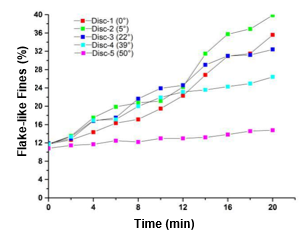Fig. 13. The flake-like fines of the different pulp samples obtained by different plates over time

Fiber external fibrillation

Fibrillation, including external and internal fibrillation, is one of the indispensable refining effects accompanied by other fiber structural changes, such as fines and fiber cutting. External fibrillation is defined as the peeling off of fibrils from the fiber surface, while leaving the fibrils attached to the fiber surface (Ebeling 1980; Page 1989). External fibrillation can be measured and studied by specific surface area (Campbell 1947; Sundström et al. 1993; Paavilainen 1994) and by microscopic imaging (Kurhila 2005; Fernando and Daniel 2004). However, the most accurate measurement comes from combining the measurement of specific surface area and fiber surface morphology. In this research, the external fibrillation was measured using a fiber quality analyzer, indicating the projection area of fibrils in relation to the projection area of the entire object, scaled into a percentage. As shown in Fig. 14, the degree of external fibrillation of the different samples refined by different refining plates increased constantly as the refining time increased—likely due to the nature of continuous refining. The maximum rate of change of the fiber external fibrillation was obtained by the pulp refined by the plate with a bar angle of 5º, and the rate of change of pulp fibrillation began to lessen when the bar angle was larger than 5º. Similarly, to the change of fiber length, a critical static attack angle existed for distinguishing the change of fiber external fibrillation, which was consistent with the curve shown previously in Fig. 10. A reasonable bar angle and static attack angle should be determined by considering the comprehensive impact on pulp and fiber properties.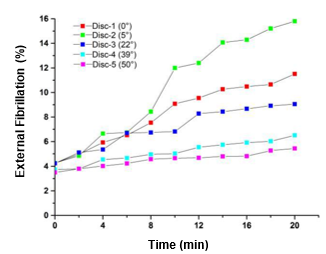Fig. 14. The fiber external fibrillation of the different pulp samples obtained by different plates over time

Paper Properties

Tensile index and tear index are two main physical strength properties widely used in paper properties analysis, and both of them are strongly affected by pulp and fiber properties and indirectly affected by the bar profile of the refiner plate.

Tensile index

The tensile index of handsheets made by pulp samples refined by different plates was analyzed, as shown in Fig. 15. As demonstrated in a previous study, the tensile index of paper is mainly dependent on the fiber bonding force and fiber length (Paavilainen 1993). And there was a critical refining time for most of refiner plates tested, except for the refining with Disc-5, in which the tensile index of the formed paper increased to a maximum value and then it decreased as the refining time increased, which is consistent with previous studies (He 2010).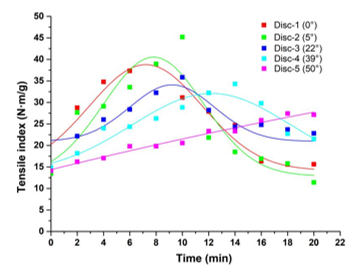Fig. 15. The tensile index of formed paper made by different pulp samples refined by different plates over time

During the beginning of refining, the fibers quickly swelled, and less fiber cutting was produced, which led to higher bonding force among fibers. The effect was a rapid increase of the tensile index of the handsheets. After the maximum value of tensile index at the critical refining time was achieved, the fiber bonding force continuously increased due to the fiber swell and fibrillation; however, the fibers were gradually shortened—which was the main factor affecting the tensile index in the latter stages of refining and thus the tensile index of paper decreased due to over-refining. The critical refining time for four different plates were 6 min, 8 min, 9.5 min, and 13 min, as shown in Fig. 15. This indicated both that the refining efficiency of refining process gradually decreased with increasing bar angle and that the most efficient one was the plate with bar angle of 0º when considering only the refining time. However, the noise is largest during the refining with Disc-1, which means that the design of plate bar angle should comprehensively consider the refining quality and noise of refining.

Figure 16 shows the fiber morphology of handsheets made by the raw material and pulp samples refined by the straight bar plates with different bar angles when the refining time was 8 min. The pulp refined by Disc-1, Disc-2, and Disc-3 demonstrated lower freeness and fiber external fibrillation, and bonding performance of handsheets made by these pulp samples was good compared to the others, which led to a higher tensile index. However, the fiber morphology of the handsheets made by pulp samples refined by Disc-4 and Disc-5 showed little change compared to that of the raw material due to its lower refining efficiency. Through the analysis above, the static attack angle should be selected in the range of approximately 45º to 70º, considering the refining efficiency and the plate wear, which can be represented by the noise of refining indirectly , and the bar angle of straight bar plates was 2.5º to 15º when the field angle was 40º.